Updating search results...

# 63 Results

View
Selected filters:
• MCCRS.Math.Content.6.SP.B.4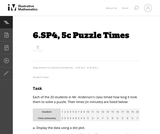Unrestricted Use
CC BY
Rating
0.0 stars

This is a task from the Illustrative Mathematics website that is one part of a complete illustration of the standard to which it is aligned. Each task has at least one solution and some commentary that addresses important asects of the task and its potential use. Here are the first few lines of the commentary for this task: Each of the 20 students in Mr. Anderson's class timed how long it took them to solve a puzzle. Their times (in minutes) are listed below: Student123456...

Subject:
Mathematics
Material Type:
Activity/Lab
Provider:
Illustrative Mathematics
Provider Set:
Illustrative Mathematics
Author:
Illustrative Mathematics
05/22/2013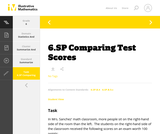Unrestricted Use
CC BY
Rating
0.0 stars

This is a task from the Illustrative Mathematics website that is one part of a complete illustration of the standard to which it is aligned. Each task has at least one solution and some commentary that addresses important aspects of the task and its potential use.

Subject:
Statistics and Probability
Material Type:
Activity/Lab
Provider:
Illustrative Mathematics
Provider Set:
Illustrative Mathematics
Author:
Illustrative Mathematics
08/06/2015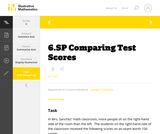Unrestricted Use
CC BY
Rating
0.0 stars

This is a task from the Illustrative Mathematics website that is one part of a complete illustration of the standard to which it is aligned. Each task has at least one solution and some commentary that addresses important aspects of the task and its potential use.

Subject:
Statistics and Probability
Material Type:
Activity/Lab
Provider:
Illustrative Mathematics
Provider Set:
Illustrative Mathematics
Author:
Illustrative Mathematics
08/06/2015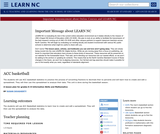Conditional Remix & Share Permitted
CC BY-NC-SA
Rating
0.0 stars

The students will use ACC basketball statistics to practice the process of converting fractions to decimals then to percents and will learn how to create and edit a spreadsheet. They will then use this spreadsheet to analyze their data. This unit is done during the basketball season which takes approximately 15 weeks from the middle of November to the middle of March. Teachers must have Clarisworks to open the sample spreadsheet in the lesson, but may recreate it in another spreadsheet program.

Subject:
Statistics and Probability
Material Type:
Lesson Plan
Provider:
University of North Carolina at Chapel Hill School of Education
Provider Set:
LEARN NC Lesson Plans
Author:
Susan Dougherty
07/14/2000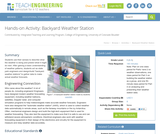Educational Use
Rating
0.0 stars

Students use their senses to describe what the weather is doing and predict what it might do next. After gaining a basic understanding of weather patterns, students act as state park engineers and design/build "backyard weather stations" to gather data to make actual weather forecasts.

Subject:
Engineering
Atmospheric Science
Material Type:
Activity/Lab
Provider:
TeachEngineering
Provider Set:
TeachEngineering
Author:
Janet Yowell
Lauren Cooper
Malinda Schaefer Zarske
10/14/2015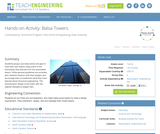Educational Use
Rating
0.0 stars

Students groups use balsa wood and glue to build their own towers using some of the techniques they learned from the associated lesson. While general guidelines are provided, give students freedom with their designs and encourage them to implement what they have learned about structural engineering. The winning team design is the tower with the highest strength-to-weight ratio.

Subject:
Architecture and Design
Engineering
Material Type:
Activity/Lab
Provider:
TeachEngineering
Provider Set:
TeachEngineering
Author:
Benjamin Burnham
Kelly Devereaux
09/18/2014Educational Use
Rating
0.0 stars

Using gumdrops and toothpicks, students conduct a large-group, interactive ozone depletion model. Students explore the dynamic and competing upper atmospheric roles of the protective ozone layer, the sun's UV radiation and harmful human-made CFCs (chlorofluorocarbons).

Subject:
Engineering
Atmospheric Science
Material Type:
Activity/Lab
Provider:
TeachEngineering
Provider Set:
TeachEngineering
Author:
Amy Kolenbrander
Denise Carlson
Janet Yowell
Malinda Schaefer Zarske
Natalie Mach
Tom Rutkowski
Tyman Stephens
10/14/2015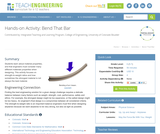Educational Use
Rating
0.0 stars

Students learn about material properties, and that engineers must consider many different materials properties when designing. This activity focuses on strength-to-weight ratios and how sometimes the strongest material is not always the best material.

Subject:
Engineering
Physics
Material Type:
Activity/Lab
Provider:
TeachEngineering
Provider Set:
TeachEngineering
Author:
Alex Conner
Geoffrey Hill
Janet Yowell
Malinda Schaefer Zarske
Tom Rutkowski
10/14/2015Educational Use
Rating
0.0 stars

Students examine the structure and function of the human eye, learning some amazing features about our eyes, which provide us with sight and an understanding of our surroundings. Students also learn about some common eye problems and the biomedical devices and medical procedures that resolve or help to lessen the effects of these vision deficiencies, including vision correction surgery.

Subject:
Engineering
Anatomy/Physiology
Material Type:
Activity/Lab
Lesson Plan
Provider:
TeachEngineering
Provider Set:
TeachEngineering
Author:
Denise W. Carlson
Lesley Herrmann
Malinda Schaefer Zarske
William Surles
09/18/2014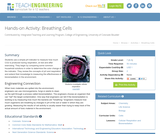Educational Use
Rating
0.0 stars

Students use a simple pH indicator to measure how much CO2 is produced during respiration, at rest and after exercising. They begin by comparing some common household solutions in order to determine the color change of the indicator. They review the concepts of pH and respiration and extend their knowledge to measuring the effectiveness of bioremediation in the environment.

Subject:
Engineering
Chemistry
Material Type:
Activity/Lab
Provider:
TeachEngineering
Provider Set:
TeachEngineering
Author:
Janet Yowell
Kaelin Cawley
Malinda Schaefer Zarske
10/14/2015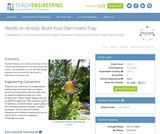Educational Use
Rating
0.0 stars

Students design and construct devices to trap insects that are present in the area around the school. The objective is to ask the right design questions and conduct the right tests to determine if the traps work .

Subject:
Engineering
Geology
Material Type:
Activity/Lab
Provider:
TeachEngineering
Provider Set:
TeachEngineering
09/18/2014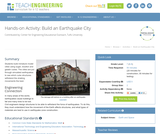Educational Use
Rating
0.0 stars

Students build miniature model cities using sugar, bouillon and gelatin cubes. The cities are put through simulated earthquakes to see which cube structures withstand the shaking movements the best.

Subject:
Engineering
Material Type:
Activity/Lab
Provider:
TeachEngineering
Provider Set:
TeachEngineering
09/18/2014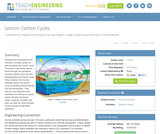Educational Use
Rating
0.0 stars

Students are introduced to the concept of energy cycles by learning about the carbon cycle. They will learn how carbon atoms travel through the geological (ancient) carbon cycle and the biological/physical carbon cycle. Students will consider how human activities have disturbed the carbon cycle by emitting carbon dioxide into the atmosphere. They will discuss how engineers and scientists are working to reduce carbon dioxide emissions. Lastly, students will consider how they can help the world through simple energy conservation measures.

Subject:
Engineering
Atmospheric Science
Material Type:
Activity/Lab
Lesson Plan
Provider:
TeachEngineering
Provider Set:
TeachEngineering
Author:
Janet Yowell
Lauren Cooper
Malinda Schaefer Zarske
09/18/2014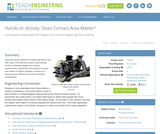Educational Use
Rating
0.0 stars

Using the same method for measuring friction that was used in the previous lesson (Discovering Friction), students design and conduct experiments to determine if the amount of area over which an object contacts a surface it is moving across affects the amount of friction encountered.

Subject:
Engineering
Physics
Material Type:
Activity/Lab
Provider:
TeachEngineering
Provider Set:
TeachEngineering
Author:
Mary R. Hebrank
10/14/2015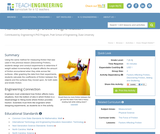Educational Use
Rating
0.0 stars

Using the same method for measuring friction that was used in the previous lesson (Discovering Friction), students design and conduct an experiment to determine if weight added incrementally to an object affects the amount of friction encountered when it slides across a flat surface. After graphing the data from their experiments, students can calculate the coefficients of friction between the object and the surface it moved upon, for both static and kinetic friction.

Subject:
Engineering
Measurement and Data
Material Type:
Activity/Lab
Lesson Plan
Provider:
TeachEngineering
Provider Set:
TeachEngineering
Author:
Mary R. Hebrank
09/26/2008Educational Use
Rating
0.0 stars

Students utilize data tables culled from the US DOE Energy Information Agency to create graphs that illustrate what types of energy we use and how we use it. An MS Excel workbook with several spreadsheets of data is provided. Students pick (or the teacher assigns) one of the data tables from which students create plots and interpret the information provided. Student groups share with the class their interpretations and new perspectives on energy resources and use.

Subject:
Engineering
Environmental Science
Material Type:
Activity/Lab
Provider:
TeachEngineering
Provider Set:
TeachEngineering
Author:
Jan DeWaters
Susan Powers
09/18/2014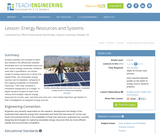Educational Use
Rating
0.0 stars

Several activities are included to teach and research the differences between renewable and non-renewable resources and various energy resources. The students work with a quantitative, but simple model of energy resources to show how rapidly a finite, non-renewable energy sources can be depleted, whereas renewable resources continue to be available. The students then complete a homework assignment or a longer, in-depth research project to learn about how various technologies that capture energy resources for human uses and their pros and cons. Fact sheets are included to help students get started on their investigation of their assigned energy source.

Subject:
Engineering
Environmental Science
Material Type:
Activity/Lab
Lesson Plan
Provider:
TeachEngineering
Provider Set:
TeachEngineering
Author:
Jan DeWaters
Susan Powers
09/18/2014Educational Use
Rating
0.0 stars

In this video segment from Cyberchase, the CyberSquad uses a graph to estimate how much the water level will rise in the Sensible Flats reservoir.

Subject:
Mathematics
Material Type:
Lecture
Provider:
PBS LearningMedia
Provider Set:
PBS Learning Media: Multimedia Resources for the Classroom and Professional Development
Author:
U.S. Department of Education
WNET
07/24/2008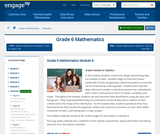Conditional Remix & Share Permitted
CC BY-NC-SA
Rating
0.0 stars

In this module, students move from simply representing data into analysis of data.  Students begin to think and reason statistically, first by recognizing a statistical question as one that can be answered by collecting data.  Students learn that the data collected to answer a statistical question has a distribution that is often summarized in terms of center, variability, and shape.  Throughout the module, students see and represent data distributions using dot plots and histograms.  They study quantitative ways to summarize numerical data sets in relation to their context and to the shape of the distribution.  As the module ends, students synthesize what they have learned as they connect the graphical, verbal, and numerical summaries to each other within situational contexts, culminating with a major project.

Subject:
Statistics and Probability
Material Type:
Module
Provider:
New York State Education Department
Provider Set:
EngageNY
02/01/2014Rating
0.0 stars

Explore how the Earth's atmosphere affects the energy balance between incoming and outgoing radiation. Using an interactive model, adjust realistic parameters such as how many clouds are present or how much carbon dioxide is in the air, and watch how these factors affect the global temperature.

Subject:
Education
Life Science
Ecology
Forestry and Agriculture
Chemistry
Physics
Material Type:
Activity/Lab
Data Set
Diagram/Illustration
Provider:
Concord Consortium
Provider Set:
Concord Consortium Collection
Author:
The Concord Consortium
12/13/2011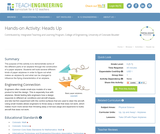Educational Use
Rating
0.0 stars

The purpose of this activity is to demonstrate some of the different parts of an airplane through the construction of a paper airplane. Students will build several different kinds of paper airplanes in order to figure out what makes an airplane fly and what can be changed to influence the flying characteristics of an airplane.

Subject:
Engineering
Material Type:
Activity/Lab
Provider:
TeachEngineering
Provider Set:
TeachEngineering
Author:
Alex Conner
Geoffrey Hill
Janet Yowell
Malinda Schaefer Zarske
Tom Rutkowski
10/14/2015Educational Use
Rating
0.0 stars

Students review the electrical appliances used at home and estimate the energy used for each. The results can help to show the energy hogs that could benefit from conservation or improved efficiency.

Subject:
Engineering
Environmental Science
Material Type:
Activity/Lab
Provider:
TeachEngineering
Provider Set:
TeachEngineering
Author:
Jan DeWaters
Susan Powers
09/18/2014Educational Use
Rating
0.0 stars

Students complete three different activities to evaluate the energy consumption in a household and explore potential ways to reduce that consumption. The focus is on conservation and energy efficient electrical devices and appliances. The lesson reinforces the relationship between power and energy and associated measurements and calculations required to evaluate energy consumption. The lesson provides the students with more concrete information for completing their culminating unit assignment.

Subject:
Engineering
Environmental Science
Material Type:
Activity/Lab
Lesson Plan
Provider:
TeachEngineering
Provider Set:
TeachEngineering
Author:
Jan DeWaters
Susan Powers
09/18/2014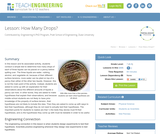Educational Use
Rating
0.0 stars

In this lesson and its associated activity, students conduct a simple test to determine how many drops of each of three liquids can be placed on a penny before spilling over. The three liquids are water, rubbing alcohol, and vegetable oil; because of their different surface tensions, more water can be piled on top of a penny than either of the other two liquids. However, this is not the main point of the activity. Instead, students are asked to come up with an explanation for their observations about the different amounts of liquids a penny can hold. In other words, they are asked to make hypotheses that explain their observations, and because middle school students are not likely to have prior knowledge of the property of surface tension, their hypotheses are not likely to include this idea. Then they are asked to come up with ways to test their hypotheses, although they do not need to actually test their hypotheses. The important points for students to realize are that 1) the tests they devise must fit their hypotheses, and 2) the hypotheses they come up with must be testable in order to be useful.

Subject:
Engineering
Physics
Material Type:
Activity/Lab
Lesson Plan
Provider:
TeachEngineering
Provider Set:
TeachEngineering
Author:
Mary R. Hebrank
09/18/2014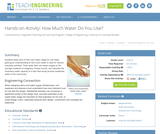Educational Use
Rating
0.0 stars

Students keep track of their own water usage for one week, gaining an understanding of how much water is used for various everyday activities. They relate their own water usages to the average residents of imaginary Thirsty County, and calculate the necessary water capacity of a dam that would provide residential water to the community.

Subject:
Engineering
Hydrology
Material Type:
Activity/Lab
Provider:
TeachEngineering
Provider Set:
TeachEngineering
Author:
Denali Lander
Denise W. Carlson
Kristin Field
Megan Podlogar
Sara Born
Tom Rutkowski
09/18/2014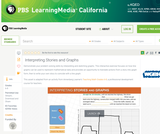Educational Use
Rating
0.0 stars

In this interactive activity adapted from Annenberg Learner's Teaching Math Grades 6&ndash;8, explore some of the ways graphs can represent mathematical data contained in a story.

Subject:
Mathematics
Material Type:
Interactive
Provider:
PBS LearningMedia
Provider Set:
PBS Learning Media Common Core Collection
Author:
U.S. Department of Education
WGBH Educational Foundation
06/20/2012Educational Use
Rating
3.0 stars

The CyberSquad creates a bar graph representing the amount of bugs that are infecting the Cybrary in this video from Cyberchase.

Subject:
Mathematics
Material Type:
Lecture
Provider:
PBS LearningMedia
Provider Set:
PBS Learning Media: Multimedia Resources for the Classroom and Professional Development
Author:
U.S. Department of Education
WNET
09/11/2008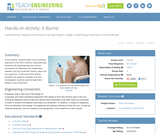Educational Use
Rating
0.0 stars

In this activity, students learn how to prevent exposure to the Sun's harmful ultraviolet rays. Students will systematically test various sunscreens to determine the relationship between spf (sun protection factor) value and sun exposure. At the end of the activity, students are asked to consider how this investigation could be used to help them design a new sunscreen.

Subject:
Engineering
Atmospheric Science
Material Type:
Activity/Lab
Provider:
TeachEngineering
Provider Set:
TeachEngineering
Author:
Brian Kay
Janet Yowell
Karen King
09/18/2014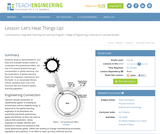Educational Use
Rating
0.0 stars

Students observe demonstrations, and build and evaluate simple models to understand the greenhouse effect, the role of increased greenhouse gas concentration in global warming, and the implications of global warming theory for engineers, themselves and the Earth. In an associated literacy activity, students learn how a bill becomes law and research global warming legislation.

Subject:
Engineering
Atmospheric Science
Material Type:
Activity/Lab
Lesson Plan
Provider:
TeachEngineering
Provider Set:
TeachEngineering
Author:
Amy Kolenbrander
Denise Carlson
Janet Yowell
Malinda Schaefer Zarske
Natalie Mach
09/18/2014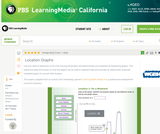Educational Use
Rating
0.0 stars

In this interactive activity adapted from Annenberg Learner&rsquo;s Teaching Math Grades 6&ndash;8, students interpret two line graphs to identify possible locations where the data might have been gathered.

Subject:
Mathematics
Material Type:
Interactive
Provider:
PBS LearningMedia
Provider Set:
PBS Learning Media Common Core Collection
Author:
U.S. Department of Education
WGBH Educational Foundation
06/20/2012Conditional Remix & Share Permitted
CC BY-NC
Rating
0.0 stars

Subject:
Mathematics
Material Type:
Full Course
Provider:
Pearson
10/06/2016Rating
0.0 stars

Distributions and Variability

Type of Unit: Project

Prior Knowledge

Students should be able to:

Represent and interpret data using a line plot.
Understand other visual representations of data.

Lesson Flow

Students begin the unit by discussing what constitutes a statistical question. In order to answer statistical questions, data must be gathered in a consistent and accurate manner and then analyzed using appropriate tools.

Students learn different tools for analyzing data, including:

Measures of center: mean (average), median, mode
Measures of spread: mean absolute deviation, lower and upper extremes, lower and upper quartile, interquartile range
Visual representations: line plot, box plot, histogram

These tools are compared and contrasted to better understand the benefits and limitations of each. Analyzing different data sets using these tools will develop an understanding for which ones are the most appropriate to interpret the given data.

To demonstrate their understanding of the concepts, students will work on a project for the duration of the unit. The project will involve identifying an appropriate statistical question, collecting data, analyzing data, and presenting the results. It will serve as the final assessment.

Subject:
Mathematics
Statistics and Probability
Material Type:
Unit of Study
Provider:
PearsonConditional Remix & Share Permitted
CC BY-NC
Rating
0.0 stars

Students calculate the mean absolute deviation (MAD) for three data sets and use it to decide which data set is best represented by the mean.The concept of mean absolute deviation (MAD) is introduced. Students understand that the sum of the deviation of the data from the mean is zero. Students calculate the MAD and understand its significance. Students find the mean and MAD of a sample set of data.Key ConceptsThe mean absolute deviation (MAD) is a measure of how much the values in a data set deviate from the mean. It is calculated by finding the distance of each value from the mean and then finding the mean of these distances.Goals and Learning ObjectivesGain a deeper understanding of mean.Understand that the mean absolute deviation (MAD) is a measure of how well the mean represents the data.Compare data sets using measures of center (mode, median, mean) and spread (range and MAD).Show that the sum of deviations from the mean is zero.

Subject:
Statistics and Probability
Material Type:
Lesson Plan
Provider:
Pearson
09/21/2015Conditional Remix & Share Permitted
CC BY-NC
Rating
0.0 stars

Students make a box plot for their typical-sixth-grader data from Lesson 7 and write a summary of what the plot shows.Using the line plot from Lesson 4, students construct a box plot. Students learn how to calculate the five-number summary and interquartile range (IQR). Students apply this knowledge to the data used in Lesson 7 and describe the data in terms of the box plot. Class discussion focuses on comparing the two graphs and what they show for the sets of data.Key ConceptsA box-and-whisker plot, or box plot, shows the spread of a set of data. It shows five key measures, called the five-number summary.Lower extreme: The smallest value in the data setLower quartile: The middle of the lower half of the data, and the value that 25% of the data fall belowMedian: The middle of the data setUpper quartile: The middle of the upper half of the data, and the value that 25% of the data are aboveUpper extreme: The greatest value in the data setThis diagram shows how these values relate to the parts of a box plot.The length of the box represents the interquartile range (IQR), which is the difference between the lower and upper quartile.A box plot divides the data into four equal parts. One quarter of the data is represented by the left whisker, two quarters by each half of the box, and one quarter by the right whisker. If one of these parts is long, the data in that quarter are spread out. If one of these quarters is short, the data in that quarter are clustered together.Goals and Learning ObjectivesLearn how to construct box plots, another tool to describe data.Learn about the five-number summary, interquartile range, and how they are related to box plots.Compare a line plot and box plot for the same set of data.

Subject:
Statistics and Probability
Material Type:
Lesson Plan
Provider:
Pearson
09/21/2015Conditional Remix & Share Permitted
CC BY-NC
Rating
0.0 stars

Students critique and improve their work on the Self Check from Lesson 13.Key ConceptsMeasures of spread (five-number summary) show characteristics of the data. It is possible to generate an appropriate data set with this information.Goals and Learning ObjectivesApply knowledge of statistics to solve problems.Identify the five-number summary, and understand measures of center and use their properties to solve problems.Track and review choice of strategy when problem solving.

Subject:
Statistics and Probability
Material Type:
Lesson Plan
Provider:
Pearson
09/21/2015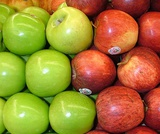Conditional Remix & Share Permitted
CC BY-NC
Rating
0.0 stars

In this lesson, students draw a line plot of a set of data and then find the mean of the data. This lesson also informally introduces the concepts of the median, or middle value, and the mode, or most common value. These terms will be formally defined in Lesson 6.Using a sample set of data, students review construction of a line plot. The mean as fair share is introduced as well as the algorithm for mean. Using the sample set of data, students determine the mean and informally describe the set of data, looking at measures of center and the shape of the data. Students also determine the middle 50% of the data.Key ConceptsThe mean is a measure of center and is one of the ways to determine what is typical for a set of data.The mean is often called the average. It is found by adding all values together and then dividing by the number of values.A line plot is a visual representation of the data. It can be used to find the mean by adjusting the data points to one value, such that the sum of the data does not change.Goals and Learning ObjectivesReview construction of a line plot.Introduce the concept of the mean as a measure of center.Use the fair-share method and standard algorithm to find the mean.

Subject:
Statistics and Probability
Material Type:
Lesson Plan
Provider:
Pearson
09/21/2015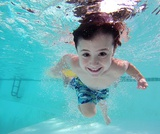Conditional Remix & Share Permitted
CC BY-NC
Rating
0.0 stars

Students make a histogram of their typical-student data and then write a summary of what the histogram shows.Students are introduced to histograms, using the line plot to build them. They investigate how the bin width affects the shape of a histogram. Students understand that a histogram shows the shape of the data, but that measures of center or spread cannot be found from the graph.Key ConceptsA histogram groups data values into intervals and shows the frequency (the number of data values) for each interval as the height of a bar.Histograms are similar to line plots in that they show the shape and distribution of a data set. However, unlike a line plot, which shows frequencies of individual data values, histograms show frequencies of intervals of values.We cannot read individual data values from a histogram, and we can't identify any measures of center or spread.Histograms sometimes have an interval with the most data values, referred to as the mode interval.Histograms are most useful for large data sets, where plotting each individual data point is impractical.The shape of a histogram depends on the chosen width of the interval, called the bin width. Bin widths that are too large or too small can hide important features of the data.Goals and Learning ObjectivesLearn about histograms as another tool to describe data.Show that histograms are used to show the shape of the data for a wider range of data.Compare a line plot and histogram for the same set of data.

Subject:
Statistics and Probability
Material Type:
Lesson Plan
Provider:
Pearson
09/21/2015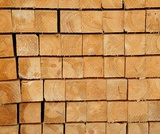Conditional Remix & Share Permitted
CC BY-NC
Rating
0.0 stars

Students use the Box Plot interactive, which allows them to create line plots and see the corresponding box plots. They use this tool to create data sets with box plots that satisfy given criteria.Students investigate how the box plot changes as the data points in the line plot are moved. Students can manipulate data points to change aspects of the box plot and to see how the line plot changes. Students create box plots that fit certain criteria.Key ConceptsThis lesson focuses on the connection between a data set and its box plot. It reinforces the idea that a box plot shows the spread of a data set, but not the individual data points.Students will observe the following similarities and differences between line plots and box plots:Line plots allow us to see and count individual values, while box plots do not.Line plots allow us to find the mean and the mode of a set of data, while box plots do not.Box plots are useful for very large data sets, while line plots are not.Box plots give us a better picture of how the values in a data set are distributed than line plots do, and they allow us to see measures of spread easily.Goals and Learning ObjectivesExperiment with different line plots to see the effect on the corresponding box plots.Create data sets with box plots that satisfy different criteria.Compare and contrast line plots and box plots.

Subject:
Statistics and Probability
Material Type:
Lesson Plan
Provider:
Pearson
09/21/2015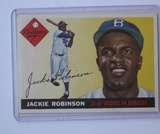Conditional Remix & Share Permitted
CC BY-NC
Rating
0.0 stars

Lesson OverviewStudents complete a card sort that requires them to match sets of statistics with the corresponding line plots.Students match cards with simple line plots to the corresponding card with measures of center. Some cards include mode, mean, median, and range, and some have one or two measures missing.  Students discuss how they determined which cards would match.Key ConceptsTo complete the card sort in this lesson efficiently, students must be able to relate statistical measures with line plots. If they start with the measures that are easy to see, they can narrow down the possible matches.The mode is the easiest measure to see immediately. It is simply the number with the tallest column of dots.The range can be found easily by subtracting the least value in the plot from the greatest.The median can be found fairly quickly by counting to the middle dot or by pairing dots on the ends until reaching the middle.The mean must be calculated by adding data values and dividing.Goals and Learning ObjectivesApply knowledge of measures of center and range to solve problems.Discuss and review strategy choices when problem solving.

Subject:
Statistics and Probability
Material Type:
Lesson Plan
Provider:
Pearson
09/21/2015Conditional Remix & Share Permitted
CC BY-NC
Rating
0.0 stars

Students will apply what they have learned in previous lessons to analyze and draw conclusions about a set of data. They will also justify their thinking based on what they know about the measures (e.g., I know the mean is a good number to use to describe what is typical because the range is narrow and so the MAD is low.).Students analyze one of the data sets about the characteristics of sixth grade students that was collected by the class in Lesson 2. Students construct line plots and calculate measures of center and spread in order to further their understanding of the characteristics of a typical sixth grade student.Key ConceptsNo new mathematical ideas are introduced in this lesson. Instead, students apply the skills they have acquired in previous lessons to analyze a data set for one attribute of a sixth grade student. Students make a line plot of the data and find the mean, median, range, MAD, and outliers. They use these results to determine a typical value for their data.Goals and Learning ObjectivesDescribe an attribute of a typical sixth grade student using line plots and measures of center (mean and median) and spread (range and MAD).Justify thinking about which measures are good descriptors of the data set.

Subject:
Statistics and Probability
Material Type:
Lesson Plan
Provider:
Pearson